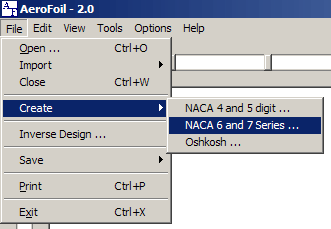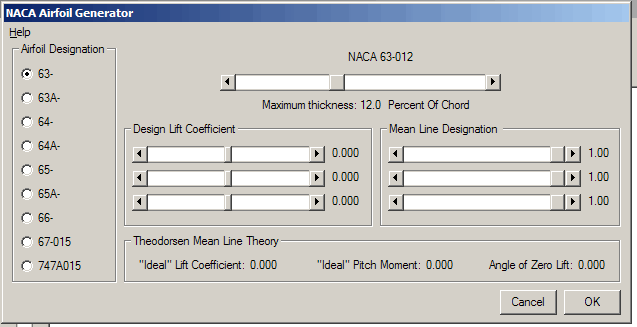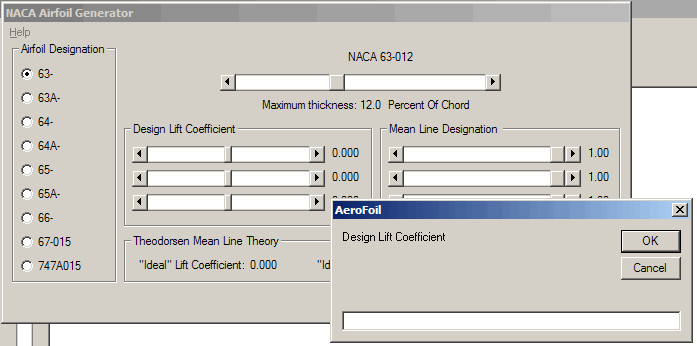## NACA 6 and 7 Series AirfoilsThe NACA 6 series airfoils were developed based on work in the late 30's and 40's to provide laminar flow over a large portion of the airfoil surface. Thickness distributions were calculated to obtain a pressure distribution that would allow laminar flow and delay the transition to turbulent. This resulted in significantly lower section drag coefficients for the six series when compared to earlier airfoils.

The thickness distributions were generally given in tabular form for thickness values of 0.06c, 0.08c, 0.09c, 0.10c, 0.12c, 0.15c, 0.18c, and 0.21c, where c is the airfoil chord. Thickness distributions based on other maximum thickness use linear interpolation to scale the profile. The 67- and 747- are later developments of this work and are only available for a 15 percent thickness.

Mean lines are based on the theory of thin wing sections, developed in the 20's. In this theory, a wing section thickness was assumed to be replaced by, and equivalent to, its mean line. The mean line was in turn composed of a chordwise distribution of vortices and a related chordwise distribution of load. Solving for the vortex strength resulted in several simple relationships between lift, drag, and pitch coefficient.

The NACA mean lines are calculated based on a design lift coefficient and a desired chordwise load distribution. The mean line designation corresponds to a load distribution that is uniform for x/c = 0 to x/c = a and linearly decreasing to zero from x/c = a to x/c = 1.

The mean line equations can be combined to alter the mean line's shape and corresponding behavior. For example, the NACA 747A315 airfoil is the 747- thickness distribution, cambered with two mean lines, a = 0.4 Cl = 0.763 and a = 0.7 Cl = -0.463. The two equations combine algebraically and result in a mean line that has a slight trailing edge reflex. The NACA 747A415 includes a third mean line of a = 1.0 Cl = 0.100.

The calculated values shown on the form for ideal angle of attack, ideal Pitch Coefficient, and angle of zero lift provide an estimate of the wing section properties, based only on the shape of the mean line. The mathematical model developed by Theodorsen is used to estimate the angle of zero lift, Pitch Coefficient, and design lift coefficient that will result from the mean line.

The mean line and thickness distributions are controlled by the slider bars and option buttons and combined to give the airfoil shape in the same manner as with the NACA 4 and 5 digit series airfoils. A maximum of three mean lines may be combined together to create the final shape.

Values used to define the thickness and camber lines may also be entered manually. Left click over the numeric value and a text box will open, type the desired value, close the window, and the value will be updated.